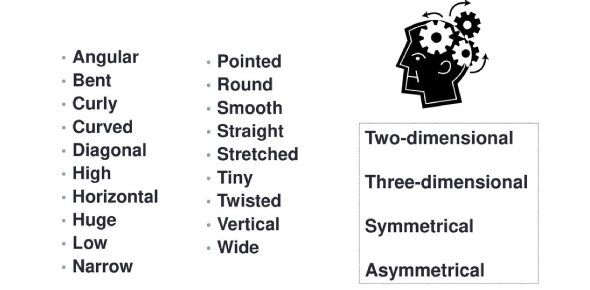# Angular Vocabulary Quiz: Test!

Approved & Edited by ProProfs Editorial Team
The editorial team at ProProfs Quizzes consists of a select group of subject experts, trivia writers, and quiz masters who have authored over 10,000 quizzes taken by more than 100 million users. This team includes our in-house seasoned quiz moderators and subject matter experts. Our editorial experts, spread across the world, are rigorously trained using our comprehensive guidelines to ensure that you receive the highest quality quizzes.
| By Treybrown
T
Treybrown
Community Contributor
Quizzes Created: 1 | Total Attempts: 1,118
Questions: 10 | Attempts: 1,118SettingsAn angle is derived from the Latin word angulus, which means "corner. "In geometrical terms, an angle is used for identifying the measurement of an angle or of a rotation, which is formed by two rays known as the sides of the angle, sharing a general endpoint, known as the vertex of the angle. This quiz has been developed to test your knowledge about Angles and Vocabulary. Let's try it out!

• 1.

• A.

An angle

• B.

A type of angle that uses varibles

• C.

An operation on 2 or more numbers to find a sum

• D.

A drawing that shows information

C. An operation on 2 or more numbers to find a sum
Explanation
Addition is an operation on 2 or more numbers to find a sum. It involves combining numbers together to calculate the total value. This process is commonly used in mathematics to find the total of multiple quantities.

Rate this question:

• 2.

### An angle of fewer than 90 degrees.

• A.

Acute angle

• B.

Obtuse angle

• C.

Right angle

• D.

Vertical angle

A. Acute angle
Explanation
An acute angle is an angle that measures less than 90 degrees. It is smaller than a right angle and an obtuse angle. A right angle measures exactly 90 degrees, while an obtuse angle measures more than 90 degrees. Therefore, an acute angle is the correct answer because it fits the given definition of an angle that is fewer than 90 degrees.

Rate this question:

• 3.

### An angle of more than 90 degrees.

• A.

Right angle

• B.

Linear angle

• C.

Obtuse angle

• D.

Straight

C. Obtuse angle
Explanation
An obtuse angle is an angle that measures more than 90 degrees. It is larger than a right angle, which measures exactly 90 degrees. A linear angle is a straight angle, measuring exactly 180 degrees. Therefore, the correct answer in this case is obtuse angle, as it is the only option that describes an angle that is greater than 90 degrees.

Rate this question:

• 4.

### The two angles together make 180 degrees.

• A.

Acute angle

• B.

Obtuse angle

• C.

Supplementary angle

• D.

C. Supplementary angle
Explanation
Supplementary angles are a pair of angles that add up to 180 degrees. In this case, the given statement suggests that the two angles being referred to are supplementary angles. This means that when the two angles are combined, their sum will equal 180 degrees.

Rate this question:

• 5.

### An angle exactly 90 degrees.

• A.

Supplementary angle

• B.

Obtuse angle

• C.

Right angle

• D.

Acute angle

A. Supplementary angle
• 6.

### Congruent angles formed by 2 lines.

• A.

• B.

Vertical angle

• C.

Straight angle

• D.

Complementary angle

B. Vertical angle
Explanation
Vertical angles are formed when two lines intersect. They are opposite angles that are congruent to each other. In other words, they have the same measure. Therefore, the correct answer is vertical angle.

Rate this question:

• 7.

### Angles with opposite rays that form 180 degrees.

• A.

Linear pair angle

• B.

Straight angle

• C.

Right angle

• D.

Acute angle

A. Linear pair angle
Explanation
A linear pair angle refers to two adjacent angles that are formed by two intersecting lines. These angles share a common vertex and a common side, while their other sides are opposite rays. The sum of the measures of these angles is always 180 degrees. Therefore, a linear pair angle is the correct answer because it is the only option that fits the given description of angles with opposite rays that form 180 degrees.

Rate this question:

• 8.

### An angle exactly 180 degrees.

• A.

Straight angle

• B.

Acute angle

• C.

Obtuse angle

• D.

Right angle

A. Straight angle
Explanation
A straight angle is formed when two lines are opposite to each other and create a line, resulting in a 180-degree angle. It is called a straight angle because the lines are in a straight line. This type of angle is the largest possible angle and is neither acute (less than 90 degrees), obtuse (greater than 90 degrees), nor a right angle (exactly 90 degrees).

Rate this question:

• 9.

### Two angles that are next to each other.(share a side)

• A.

Obtuse Angle

• B.

Linear pair angle

• C.

• D.

Vertical angle

Explanation
Adjacent angles are two angles that share a common side and a common vertex. In other words, they are side by side and do not overlap. In this case, the given correct answer is "Adjacent angle" because it accurately describes the relationship between two angles that are next to each other and share a side.

Rate this question:

• 10.

### Divides angle into 2 congruent angles.

• A.

Right angle

• B.

Angle bisector

• C.

Straight angle

• D.

Obtuse angle

B. Angle bisector
Explanation
An angle bisector is a line or ray that divides an angle into two congruent angles. In other words, it splits the angle into two equal parts. Therefore, the given correct answer is "angle bisector".

Rate this question:

Related TopicsBack to top